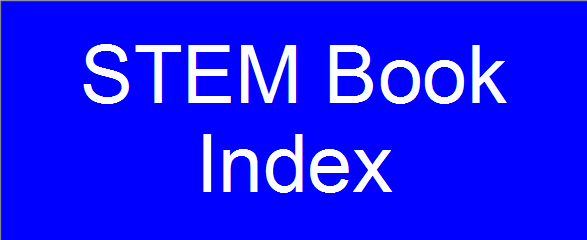On this webpage you will find my solutions to the second edition of "Nonlinear Dynamics and Chaos with Applications to Physics, Biology, Chemistry, and Engineering" by Steven H. Strogatz. Here is a link to the book's page on amazon.com. If you find my work useful, please consider making a donation. Thank you.

• Chapter 2: Flows on the Line
• Section 2.1: A Geometric Way of Thinking
• Section 2.2: Fixed Points and Stability
• Section 2.3: Population Growth
• Section 2.4: Linear Stability Analysis
• Section 2.5: Existence and Uniqueness
• Section 2.6: Impossibility of Oscillations
• Section 2.7: Potentials
• Section 2.8: Solving Equations on the Computer

• Chapter 3: Bifurcations
• Section 3.2: Transcritical Bifurcation
• Section 3.3: Laser Threshold
• Section 3.4: Pitchfork Bifurcation
• Section 3.5: Overdamped Bead on a Rotating Hoop
• Section 3.6: Imperfect Bifurcations and Catastrophes
• Section 3.7: Insect Outbreak

• Chapter 4: Flows on the Circle
• Section 4.1: Examples and Definitions
• Section 4.2: Uniform Oscillator
• Section 4.3: Nonuniform Oscillator
• Section 4.4: Overdamped Pendulum
• Section 4.5: Fireflies
• Section 4.6: Superconducting Josephson Junctions
• Chapter 5: Linear Systems
• Section 5.1: Definitions and Examples
• Section 5.2: Classification of Linear Systems
• Section 5.3: Love Affairs

• Chapter 6: Phase Plane
• Section 6.1: Phase Portraits
• Section 6.2: Existence, Uniqueness, and Topological Consequences
• Section 6.3: Fixed Points and Linearization
• Section 6.4: Rabbits versus Sheep
• Section 6.5: Conservative Systems
• Section 6.6: Reversible Systems
• Section 6.7: Pendulum
• Section 6.8: Index Theory

• Chapter 7: Limit Cycles
• Section 7.1: Examples
• Section 7.2: Ruling Out Closed Orbits
• Section 7.3: Poincaré-Bendixson Theorem
• Section 7.4: Liénard Systems
• Section 7.5: Relaxation Oscillations
• Section 7.6: Weakly Nonlinear Oscillators

• Chapter 8: Bifurcations Revisited
• Section 8.1: Saddle-Node, Transcritical, and Pitchfork Bifurcations
• Section 8.2: Hopf Bifurcations
• Section 8.3: Oscillating Chemical Reactions
• Section 8.4: Global Bifurcations of Cycles
• Section 8.5: Hysteresis in the Driven Pendulum and Josephson Junction
• Section 8.6: Coupled Oscillators and Quasiperiodicity
• Section 8.7: Poincaré Maps
• Chapter 9: Lorenz Equations
• Section 9.1: A Chaotic Waterwheel
• Section 9.2: Simple Properties of the Lorenz Equations
• Section 9.3: Chaos on a Strange Attractor
• Section 9.4: Lorenz Map
• Section 9.5: Exploring Parameter Space
• Section 9.6: Using Chaos to Send Secret Messages

• Chapter 10: One-Dimensional Maps
• Section 10.1: Fixed Points and Cobwebs
• Section 10.2: Logistic Map: Numerics
• Section 10.3: Logistic Map: Analysis
• Section 10.4: Periodic Windows
• Section 10.5: Liapunov Exponent
• Section 10.6: Universality and Experiments
• Section 10.7: Renormalization

• Chapter 11: Fractals
• Section 11.1: Countable and Uncountable Sets
• Section 11.2: Cantor Set
• Section 11.3: Dimension of Self-Similar Fractals
• Section 11.4: Box Dimension
• Section 11.5: Pointwise and Correlation Dimensions

• Chapter 12: Strange Attractors
• Section 12.1: The Simplest Examples
• Section 12.2: Hénon Maps
• Section 12.3: Rössler Maps
• Section 12.4: Chemical Chaos and Attractor Reconstruction
• Section 12.5: Forced Double-Well Oscillator
Section 2.1 Section 2.2 Section 2.3 Section 2.4
Problem 2.1.1 Problem 2.2.1 Problem 2.3.1 Problem 2.4.1
Problem 2.1.2 Problem 2.2.2 Problem 2.3.2 Problem 2.4.2
Problem 2.1.3 Problem 2.2.3 Problem 2.3.3 Problem 2.4.3
Problem 2.1.4 Problem 2.2.4 Problem 2.3.4 Problem 2.4.4
Problem 2.1.5 Problem 2.2.5 Problem 2.3.5 Problem 2.4.5
Problem 2.2.6 Problem 2.3.6 Problem 2.4.6
Problem 2.2.7 Problem 2.4.7
Problem 2.2.8 Problem 2.4.8
Problem 2.2.9 Problem 2.4.9
Problem 2.2.10
Problem 2.2.11
Problem 2.2.12
Problem 2.2.13
Section 2.5 Section 2.6 Section 2.7 Section 2.8
Problem 2.5.1 Problem 2.6.1 Problem 2.7.1 Problem 2.8.1
Problem 2.5.2 Problem 2.6.2 Problem 2.7.2 Problem 2.8.2
Problem 2.5.3 Problem 2.7.3 Problem 2.8.3
Problem 2.5.4 Problem 2.7.4 Problem 2.8.4
Problem 2.5.5 Problem 2.7.5 Problem 2.8.5
Problem 2.5.6 Problem 2.7.6 Problem 2.8.6
Problem 2.7.7 Problem 2.8.7
Problem 2.8.8
Problem 2.8.9